# Karnaugh Maps (K-Map), Truth Tables, Boolean Expressions & Examples

## Karnaugh Maps (K-Map), 1-6 Variables Simplification & Examples

### What is Karnaugh Map (K-Map)?

Karnaugh map or K-map is a map of a function used in a technique used for minimization or simplification of a Boolean expression. It results in less number of logic gates and inputs to be used during the fabrication.

Booleans expression can be simplified using Boolean algebraic theorems but there are no specific rules to make the most simplified expression. However, K-map can easily minimize the terms of a Boolean function.

Unlike an algebraic method, K-map is a pictorial method and it does not need any Boolean algebraic theorems.

K-map is basically a diagram made up of squares. Each of these squares represents a min-term of the variables. If n = number of variables then the number of squares in its K-map will be 2n. K-map is made using the truth table. In fact, it is a special form of the truth table that is folded upon itself like a sphere. Every two adjacent squares of the k-map have a difference of 1-bit including the corners.

Karnaugh map can produce Sum of product (SOP) or product of Sum (POS) expression considering which of the two (0,1) outputs are being grouped in it. The grouping of 0’s result in Product of Sum expression & the grouping of 1’s result in Sum of Product expression. The expression produced by K-map may be the most simplified expression but not unique. There can be more than 1 simplified expression for a single function but they all perform the same.

### Grey Code

In Gray code, every two consecutive number has a difference of 1-bit. As the squares in K-map also differs from its adjacent square by 1-bit which is why the variables in K-map are written in grey code. The gray code ensures that each cell of K-map is in 1-bit difference with each other.

• You may also read: Counter and Types of Electronic Counters

#### BCD to Gray Code using K-Map

The table for BCD to Gray code is given below.### Rules of Minimization in K-Map

1. While grouping, you can make groups of 2n number where n=0, 1, 2, 3…
2. You can either make groups of 1’s or 0’s but not both.
3. Grouping of 1’s lead to Sum of Product form and Grouping of 0’s lead to Product of Sum form.
4. While grouping, the groups of 1’s should not contain any 0 and the group of 0’s should not contain any 1.
5. The function output for 0’s grouping should be complemented as F’.
6. Groups can be made vertically and horizontally but not diagonally.
7. Groups made should be as large as possible even if they overlap.
8. All the like term should be in a group even if they overlap.
9. Uppermost& lowermost squares can be made into a group together as they are adjacent (1-bit difference). Same goes for the corner squares.
10. Each group represents a term in the Boolean expression. Larger the group, smaller and simple the term.
11. The product of those literals that remains unchanged in a single group makes the term of the expression.
12. Don’t care “x” should also be included while grouping to make a larger possible group.

Karnaugh map of 2 to 4 variables is very easy. However, 5 and 6 variable K-map is a little bit complex. We will discuss one by one in details.

You may also read: Digital Flip-Flops – SR, D, JK and T Flip Flops

### 2 Variable K-Map

2 variables have 2n = 22 = 4 minterms. Therefore there are 4 cells (squares) in 2 variable K-map for each minterm.

Consider variable A & B as two variables. The rows of the columns will be represented by variable B.

The square facing the combination of the variable represents that min term as shown in fig below.Grouping in 2 variables K-map is easy as there are few squares.

#### Example of 2 Variable K-Map

Function F (A, B)F = ∑ (m0, m1, m2) = A̅B̅ +A̅B +AB̅

K-map from Truth table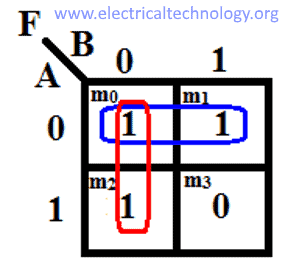We made 2 groups of 1’s. each group contains 2 minterms.

In the first group, variable A is changing & B remains unchanged. So the first term of the output expression will be B̅ (because B = 0 in this group).

In the 2nd group, Variable B is changing and variable A remains unchanged. So the second term will be of the output expression will be A̅ (because A=0 in this group).

Now the simplifies expression will be the sum of these two terms as given below,

F = A̅ + B̅

Compare this expression with the original expression of the function, this expression only uses one gate during its implementation.

### 3 Variable K-Map

3 variables make 2n=23=8 min terms, so the Karnaugh map of 3 variables will have 8 squares(cells) as shown in the figure given below.3 variable K-map can be in both forms. Note the combination of two variables in either form is written in Gray code. So the min terms will not be in a decimal order.

The uppermost & lowermost cells are adjacent in the first form of K-map, the leftmost and rightmost cells are also adjacent in the second form of K-map. So they can be made into groups.

Some examples of grouping:

You can make groups of 2, 4 & 8 cells having same 1s or 0s.Notice the groups of the uppermost & lowermost cells. They are adjacent as there is only one-bit difference. That is why they can be grouped together. Don’t make unnecessary groups. All 1s or 0s should be grouped, not all possible groups of 1s or 0s should be made.

#### Example of 3 Variable K-Map

F (A,B,C) = ∑ ( m0, m1, m2, m4, m5, m6 )This example shows that you can make the groups overlap each other to make them as large as possible and cover all the 1s.In this first group ( m0, m2, m6, m4 ), A &B are changing so we will eliminate it. However, C remains unchanged in this group. So the term this group produce will be C̅ (because C=0 in this group).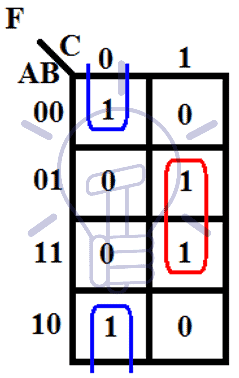In the 2nd group (m0,m1,m4,m5), A and C are changing so it will be eliminated from the term. However, B remains unchanged in this group. So the term this group produce will be B̅ (because B=0 in this group).

The sum of these two terms will make the simplified expression of the function as given below.

F = B̅ + C̅

Another example of grouping of 2 is given below. It shows how the corner min terms are grouped.

In the first group (m0,m4), A is changing. B & C remains unchanged. So the term will be B̅C̅ (B=0,C=0 in this group).

In 2nd group (m3,m7), A is changing. B & C remains unchanged. BC will be the term because B=1,C=1 in this group.

So This K-map leads to the expression

F = B̅C̅ + BC

These two examples show that a group of 4 cells give a term of 1 literal and a group of 2 cells gives a term of 2 literals and a group of 1 cell gives a term of 3 literals. So the larger the group,the smaller and simple the term gets.

### 4-variable K-Map

4 variables have 2n=24=16 minterms. So a 4-variable k-map will have 16 cells as shown in the figure given below.Each cell (min term) represent the variables in front of the corresponding row & column.

The variables are in gray code (1-bit change). The four cells of the corner are adjacent to each other as there is a1-bit difference even if they are not touching physically. So they can be grouped together.

Some example of grouping in 4-variable k-map is given below: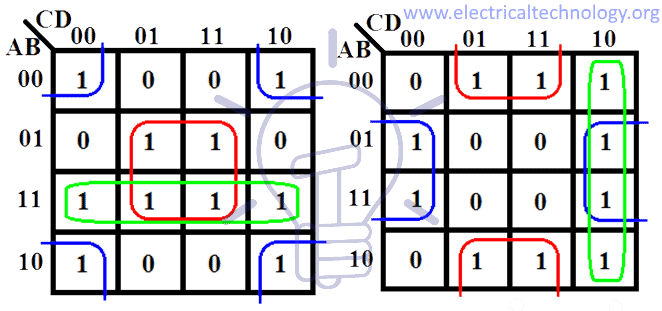As you can see in the example above the 4 corner cells make a group. In the second example, leftmost columns can be grouped with rightmost column and uppermost row with the lowermost row.

These groups should be as large as possible containing 1,2,4,8 or 16 cells. The terms of the expression depend on these groups. If the group contains:

One square, then it will give a term of 4 literals

Two squares, then it will give a term of 3 literals

Four squares, then it will give a term of 2 literals

Eight square, then it will give a term of 1 literal

Sixteen square which will cover the whole 4-variable k-map which means constant 1 output.

#### Example of 4 Variable K-Map

F(A,B,C,D) = ∑( m0, m1, m2, m4, m5, m6, m8, m9, m12, m13, m14 )First of all, try to make the biggest possible groups as shown in this example. Corner 1s can also be made into a group of 4. The remaining last 1 should be combined with the pre-made group to make a big overlapping group.

Group of 8 will give a term of 1 literal that remains unchanged i.e. C̅

Corner group of 4 will give term with 2 literals that remain unchanged i.e. B̅D̅

The last group of 4 will give A̅D̅ because they remained unchanged in the group.

So the expression will be

F =  C̅ + B̅D̅ + A̅D̅

### 5 & 6 Variable Karnaugh Maps

K-Map is used for minimization or simplification of a Boolean expression. 2-4 variable K-maps are easy to handle. However, the real challenge is 5 and 6 variable K-maps.  Visualization of 5 & 6 variable K-map is a bit difficult. When the number of variables increases, the number of the square (cells) increases. And drawing the K-map becomes a bit complicated because of drawing the adjacent cells.

### 5 Variables K-Map

5 variables have 32 min terms,which mean 5 variable karnaugh map has 32 squares (cells).

A 5-variable K-map is made using two 4-variable K-maps. Consider 5 variables A,B,C,D,E. their 5 variable K-map is given below.These both 4-variable Karnaugh map together represents a 5-variable K-map for variable A,B,C,D,E. Notice variable A over the top of each 4-variable K-map. For A=0, the left K-map is selected and right map for A = 1.

Each corresponding squares (cells) of these 2 4-variable K-maps are adjacent. Visualize these both K-maps on top of each other. m0 is adjacent to m16, so is m1 to m17 so on until the last square.

The rule (method) of grouping is same for each of the 4-variable k-maps. However, you also need to check the corresponding cells in both K-maps as well. A few example of grouping is given below.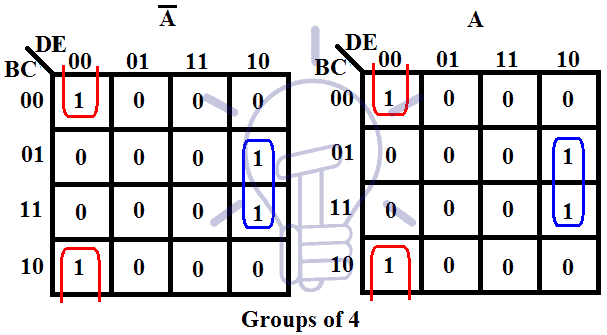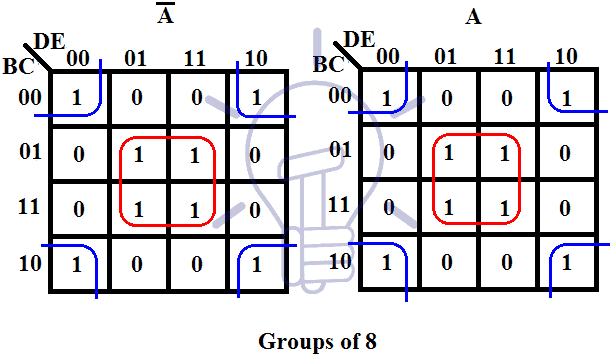In these examples, each group is differentiated using different colors.

#### Example of 5 Variables K-Map

F (A,B,C,D,E)  =  ∑  ( m0, m2, m5, m7, m8, m10, m16, m21, m23, m24, m27, m31 )This is the 5-variable k-map for the function given above. There are four groups made in this K-map. Each group has a different color to differentiate between them.

The red color group is a group of 4 min terms made between both 4-variable k-maps because they are adjacent cells and it overlaps the green group.

The yellow group is also a group of 4 min terms made between adjacent cells of the 4-variable k-maps.

The green group is a group of 4 min terms made in the left 4-variable k-map. The blue group is of 2 min-terms made in the right 4-variable k-map because there are no common adjacent cells in the other k-map.

Green color group of 4 min term will produce the term A̅C̅E̅. The individual 4-variable K-map will produce C̅E̅ as they are not changing in the group but variable A should also be taken into account because this individual 4-variable k-map is being represented by A̅.

The red color group will produce C̅D̅E̅. This group is made between both K-maps which means variable A changes and in individual K-map, B changes so these both variables will be eliminated from the term. Only C̅D̅E̅ remains unchanged in this group.

The yellow group will produce B̅CE because these literals are not changing in this group.

Blue group of 2 min terms will produce the term ABDE as they remain unchanged in this group.

The simplified expression will be the sum of these 4 terms, which is given below:

F = A̅C̅E̅ + C̅D̅E̅ + B̅CE + ABDE

You may also read: Digital Synchronous Counter – Types, Working & Applications

### 6-Variable Karnaugh Map

6-variable k-map is a complex k-map which can be drawn. Visualizing 6-variable k-map is a little bit tricky.

6 variables make 64 min terms, this means that the k-map of 6 variables will have 64 cells. Its geometry becomes difficult to draw as these cells are adjacent to each other in all direction in 3-dimensions i.e. a cell is adjacent to upper, lower, left, right, front and back cells at the same time. we will draw it like 5-variable k-map as shown in the figure below.The 6-variable k-map is made from 4-variable 4 k-maps. As you can see variable A on the left side select 2 k-maps row-wise between these 4 k-maps. A = 0 for the upper two K-maps and A = 1 for the lower two K-maps. Variable B on top of these K-maps select 2 k-maps column-wise. B = 0 for left 2 K-maps and B = 1 for right 2 K-maps.

Imagine these 4-variable K-maps as a single square, these k-maps are adjacent to each other horizontally and vertically but not diagonally because these cells have 1-bit difference. The groups between these k-maps should be made as done in 5-variable K-map but you cannot make groups between diagonal k-maps.

Some examples of grouping in 6-variable K-map are given below.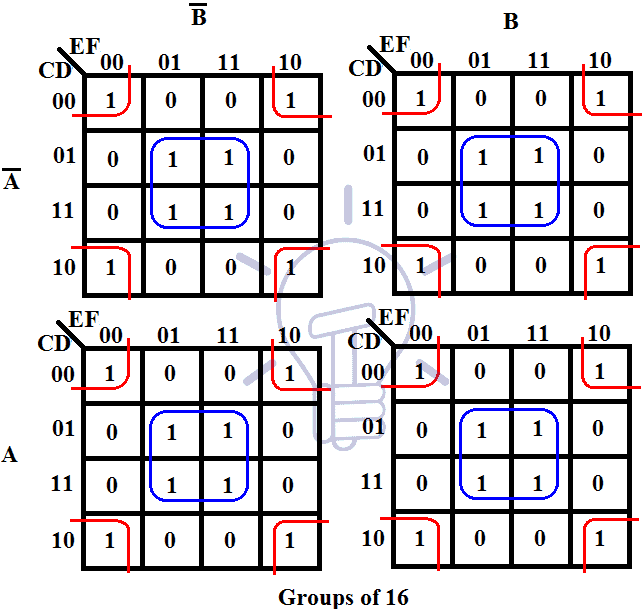Group of 16 min-terms between 4 k-maps as they are all adjacent. Visualize these k-maps on top of each other.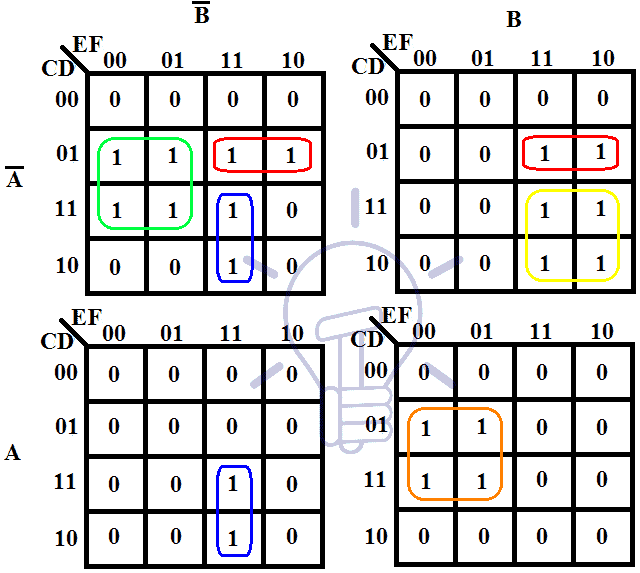In this example, there are 5 groups of 4 min-terms. Notice the min-terms in the diagonal K-maps,they make a separate group because these K-maps are not adjacent.

#### Example of 6 Variable K-Map maping

F  =  ∑ ( m0, m2, m8, m9, m10, m12, m13, m16, m18, m24, m25, m26, m29, m31, m32, m34, m35, m39, m40, m42, m43, m47, m48, m50, m56, m58, m61, m63 )

Its 6-variable K-map is given below:There are 5 groups in this K-map each colored different.

Green group is made of 16 min-terms between all 4 individual K-maps. In this group, AB keeps changing so they will be eliminated from the term. C & E are also changing so they will be eliminated from the term too. So the term will become D̅F̅ because they remain unchanged throughout the group.

Red group is made of 4 min-terms. In this group, B is changing so it will be eliminated. D is also changing. So the only remaining unchanged literals will make the term which is A̅CE̅F.

Blue group is also made of 4 min-terms. The only changing variables are DF throughout this group so they will be eliminated from the term. The non-changed literal in this group are A̅B̅CE̅ which will be the termproduced by this group.

The Yellow group is also a group of 4 min-terms and the changing variables in this group are AE. The literal that remains unchanged are BCDF in this group.

The black group is of 4 min-terms too. This group produces the term AB̅EF because they are the unchanged literals in this group.

The simplified expression of the function will be the sum of these 5 terms from these groups. The expression is given below:

F = D̅F̅ + A̅CE̅F + A̅B̅CE̅ + BCDF + AB̅EF

Tags